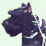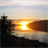# Calculating slope with NED

2886
2
07-20-2015 02:16 PMNew Contributor

I am trying to calculate, then reclassify, a DEM raster to show areas that are 20%slope and greater. I am able to calculate the slope using the slope tool in Spatial Analyst, but I am having trouble interpreting the output raster. The output raster has extremely high values and it doesn't look like the result I could then just reclassify to show 20% and greater. Am I going about this in the right fashion? Does the Z factor have something to do with this?

1 Solution

Accepted SolutionsbyMVP Honored Contributor

I'm guessing this DEM is in a geographic coordinate reference system (decimal degrees), horizontally, and meters or feet vertically. You must first use the Project tool to switch to a projected coordinate system (preferably in the same horizontal units as vertical elevation), then run Slope to get meaningful values.

2 RepliesbyMVP Honored Contributor

I'm guessing this DEM is in a geographic coordinate reference system (decimal degrees), horizontally, and meters or feet vertically. You must first use the Project tool to switch to a projected coordinate system (preferably in the same horizontal units as vertical elevation), then run Slope to get meaningful values.Occasional Contributor III

To build on what Darren said, it would be easiest if you choose a projected coordinate system with the same linear units as the units used for elevation in the DEM.  If this is not an option, here is what the tool help states about using the "Z factor" parameter:

"Number of ground x,y units in one surface z unit.

The z-factor adjusts the units of measure for the z units when they are different from the x,y units of the input surface. The z-values of the input surface are multiplied by the z-factor when calculating the final output surface.

If the x,y units and z units are in the same units of measure, the z-factor is 1. This is the default.

If the x,y units and z units are in different units of measure, the z-factor must be set to the appropriate factor, or the results will be incorrect. For example, if your z units are feet and your x,y units are meters, you would use a z-factor of 0.3048 to convert your z units from feet to meters (1 foot = 0.3048 meter)."